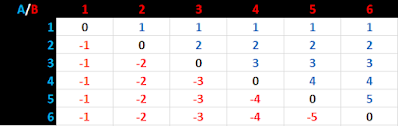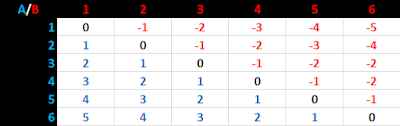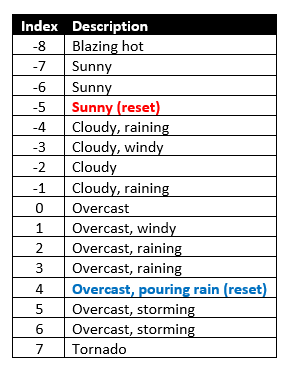### a different math for ladder tables

my friend ty (@eldritchmouse) wrote up a great blog post about a new way to introduce memory states into random tables, called ladder tables (link)! i wanted to share my thoughts on how to accomplish the same thing with slightly different math, with no reason other than to fit it to my own tastes, and in case someone else would find it useful.

the original procedure for ladder tables (assuming one where both axes use d6) is as follows, where dA represents a tendency towards one extreme of the table and dB represents the other extreme:

1. set initial index to dice dA + dB.
2. each turn, roll dA and dB again. now dA and dB represent the opposite ends of the table.
• if dA < dB, move the index down by dA.
• if dB < dA, move the index up by dB.
• if dA = dB, do not move the index up or down.

there is also the case that if the index exceeds the maximum (or minimum), there is a more moderate index to which it resets.

the results in a move table that looks like this:i suggest a different procedure:

1. set initial index to dA - dB (optional).
2. each turn, roll dA dB. and change the index by this amount.

which looks like this:when comparing the two tables, you can see that the rate of change of the index is equivalent! the main difference is really that the higher die indicates the direction of change, which you can see reflected in the tables above. this means that in a way, the table is flipped (as you can see by how the results change on either half of the zero-line switch color).

to me, this has the following benefits:

• the initial state is most likely to be indexed zero.
• the dice are consistent in representing positive or negative change.
• the die with the highest roll corresponds with the direction of the index change.
• the subtraction of dA - dB encompasses all the cases of the original comparative algorithm, including the zero result where dA = dB.

the main change necessary, and this is really optional, is to index the table from -5 to +5 (when using two six-sided dice). that way the two dice A and B are consistent in their meaning from the beginning of their use. this has the handy effect that the most likely initial index is 0, representing a balance of the two extremes.

an example of these new indexing is as follows, using the table ty wrote in his blog post (the original table has 8 entries indexed < 9 and 7 entries indexed > 9, and uses dA = d8 and dB = d8):the easy way to convert is to subtract the average initial index from each index, such that the middle average result becomes zero.

1.I have not at all thought through the probabilities or other particulars of this idea yet, but on-face, this seems very similar conceptually to the Hex Flower. I'm sure if I spent a moment to think it through I could answer my own question, but it may still be useful to ask, are there any probabilistic differences or more fundamental differences between this and Hex Flower? Could they be translatable and would there be any advantage to doing so? Again just on-face, my instinct is that Hex Flower is a little more intuitive (although that could be a familiarity bias), but from a software and data analytics perspective, a table is much easier.

1.hi there! ty was inspired by the hex flower to invent ladder tables as an easier method of using tables with memory :) the probabilities and other aspects are i think fundamentally different because a table is only one-dimensional, but the basic function is the same. i have a friend who made a hex terrain determiner using a ladder table since he had difficulty with the hex flower, and he seems to be happier with it!

2.Fitting a line to a table per se I suppose is one dimensional, but you can fit a plane / n-dimensional hyperplane to a matrix / multidimensional array 🤯...

3.absolutely!! though my main worry at that point would be that the table wouldn't be human-readable, but it would be a computer's model of a hex grid 😂 maybe that would be a fun challenge, to make a 3D-simulating turing machine table out of humor and hubris.

4.If it were just a plane it could still be visualized in a reasonably coherent way, and in research science, data analytics, and ML, there are all sorts of ways to visualize these kinds of effects in ways that are human readable. Imo I think the main advantage of something like this over hex flower is that it would be much easier to do these more advanced kinds of tooling, although it is true that at that point some thought needs to go into how to visualize it.

A long time ago I actually was working on an object oriented data abstraction for hex mapping to do similar kinds of tooling in both Cartesian and axial space (if I'm remembering correctly...), then sort of lost track of it but I should get back to it some day...

5.I used this site for reference for some of the underlying math and algorithms but was building my own data abstraction on top of it.
https://www.redblobgames.com/grids/hexagons/implementation.html